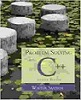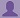SHARE

To start with, Sr2Jr’s first step is to reduce the expenses related to education. To achieve this goal Sr2Jr organized the textbook’s question and answers. Sr2Jr is community based and need your support to fill the question and answers. The question and answers posted will be available free of cost to all.# Problem Solving With C++

Authors: Walter Savitch ,julia Lobur Chapter: More Flow Of Control
ISBN: 9780321531346 Exercise: Self-test Exercises
Edition: 7 Question: 1
 Previous Next

###Question

Determine the value, true or false, of each of the following Boolean expressions, assuming that the value of the variable count is 0 and the value of the variable limit is 10. Give your answer as one of the values true or false.

a. (count == 0) && (limit < 20)

b. count == 0 && limit < 20

c. (limit > 20) || (count < 5)

d. !(count == 12)

e. (count == 1) && (x < y)

f. (count < 10) || (x < y)

g. !( ((count < 10) || (x < y)) && (count >= 0) )

h. ((limit/count) > 7) || (limit < 20)

i. (limit < 20) || ((limit/count) > 7)

j. ((limit/count) > 7) && (limit < 0)

k. (limit < 0) && ((limit/count) > 7)

l. (5 && 7) + (!6)

###Answer

1. True

2. True

3. True

4. True

5. False

6. True

7. False

8. An error message produced

9. True

10. An error message produced

11. False

### Discussions

Post the discussion to improve the above solution.